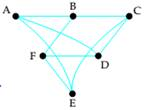# Use Dirac's theorem to verify that the graph is Hamiltonian. Then find a Hamiltonian circuit.### Mathematical Excursions (MindTap C...

4th Edition
Richard N. Aufmann + 3 others
Publisher: Cengage Learning
ISBN: 9781305965584

#### Solutions

Chapter
Section### Mathematical Excursions (MindTap C...

4th Edition
Richard N. Aufmann + 3 others
Publisher: Cengage Learning
ISBN: 9781305965584
Chapter 5.2, Problem 4ES
Textbook Problem
1 views

## Use Dirac's theorem to verify that the graph is Hamiltonian. Then find a Hamiltonian circuit.To determine

To determine if the graph is Hamiltonian using Dirac's theorem. Then to find a Hamiltonian circuit.

### Explanation of Solution

Given information:

Given, graph is:

Calculation:

Dirac's theorem states, for a connected graph with at least three vertices and no multiple edges, if the number of vertices is n and every vertex has degree of at least n/2, then the graph is a Hamiltonian graph.

Here,

The number of vertices in the graph is 6 (A, B, C, D, E, F)

So the graph is Hamiltonian if the degree of every vertex is at least 6/2=3

And,

The degree of the vertex A is 3

The degree of the vertex B is 3

The degree of the vertex ...

### Still sussing out bartleby?

Check out a sample textbook solution.

See a sample solution

#### The Solution to Your Study Problems

Bartleby provides explanations to thousands of textbook problems written by our experts, many with advanced degrees!

Get Started

Find more solutions based on key concepts
Find all possible real solutions of each equation in Exercises 3144. x31=0

Finite Mathematics and Applied Calculus (MindTap Course List)

In Exercises 23-32, determine whether the statement is true or false. Give a reason for your choice. 32. 52/3 ...

Applied Calculus for the Managerial, Life, and Social Sciences: A Brief Approach

Find the exact length of the curve. 20. y = 1 ex, 0 x 2

Single Variable Calculus: Early Transcendentals

Express 9712 as a mixed number.

Mathematics For Machine Technology

The horizontal asymptote(s) for f(x)=|x|x+1 is (are): a) y =0 b) y = 1 c) y = 1 d) y = 1 and y = 1

Study Guide for Stewart's Single Variable Calculus: Early Transcendentals, 8th

True or False:

Study Guide for Stewart's Multivariable Calculus, 8th

In Problems 21 and 22 solve the given initial-value problem. 22. y1/2dydx+y3/2=1, y(0) = 4

A First Course in Differential Equations with Modeling Applications (MindTap Course List)

51. In developing patient appointment schedules, a medical center wants to estimate the mean time that a staff ...

Modern Business Statistics with Microsoft Office Excel (with XLSTAT Education Edition Printed Access Card) (MindTap Course List)

Reminder Round all answers to two decimals places unless otherwise indicated. Where Lines with Different Slopes...

Functions and Change: A Modeling Approach to College Algebra (MindTap Course List)